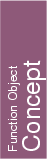## Kernel::CoplanarOrientation_3

A model for this must provide:

Orientation
 fo.operator() ( Kernel::Point_3 p, Kernel::Point_3 q, Kernel::Point_3 r, Kernel::Point_3 s)
Let P be the plane defined by the points p, q, and r. Note that the order defines the orientation of P. The function computes the orientation of points p, q, and s in P: Iff p, q, s are collinear, COLLINEAR is returned. Iff P and the plane defined by p, q, and s have the same orientation, POSITIVE is returned; otherwise NEGATIVE is returned.
Precondition: p, q, r, and s are coplanar and p, q, and r are not collinear.

Orientation
 fo.operator() ( Kernel::Point_3 p, Kernel::Point_3 q, Kernel::Point_3 r)
If p,q,r are collinear, then COLLINEAR is returned. If not, then p,q,r define a plane P. The return value in this case is either POSITIVE or NEGATIVE, but we don't specify it explicitely. However, we guarantee that all calls to this predicate over 3 points in P will return a coherent orientation if considered a 2D orientation in P.